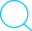汇编语言-INT和AH表

mov ah,01; int 21h;

Posted by Kingtous on March 12, 2019

AH的值需要查表取得，表在下面

​ INT 21H

AH 功能 调用参数 返回参数
00 程序终止(同INT 20H) CS=程序段前缀
01 键盘输入并回显   AL=输入字符
02 显示输出 DL=输出字符
03 异步通迅输入   AL=输入数据
04 异步通迅输出 DL=输出数据
05 打印机输出 DL=输出字符
06 直接控制台I/O DL=FF(输入) DL=字符(输出) AL=输入字符
07 键盘输入(无回显)   AL=输入字符
08 键盘输入(无回显) 检测Ctrl-Break   AL=输入字符
09 显示字符串 DS:DX=串地址 ‘\$’结束字符串
0A 键盘输入到缓冲区 DS:DX=缓冲区首地址 (DS:DX)=缓冲区最大字符数 (DS:DX+1)=实际输入的字符数
0B 检验键盘状态   AL=00 有输入 AL=FF 无输入
0C 清除输入缓冲区并 请求指定的输入功能 AL=输入功能号 (1,6,7,8,A)
0D 磁盘复位   清除文件缓冲区
0E 指定当前缺省的磁盘驱动器 DL=驱动器号 0=A,1=B,… AL=驱动器数
0F 打开文件 DS:DX=FCB首地址 AL=00 文件找到 AL=FF 文件未找到
10 关闭文件 DS:DX=FCB首地址 AL=00 目录修改成功 AL=FF 目录中未找到文件
11 查找第一个目录项 DS:DX=FCB首地址 AL=00 找到 AL=FF 未找到
12 查找下一个目录项 DS:DX=FCB首地址 (文件中带有*或?) AL=00 找到 AL=FF 未找到
13 删除文件 DS:DX=FCB首地址 AL=00 删除成功 AL=FF 未找到
14 顺序读 DS:DX=FCB首地址 AL=00 读成功 =01 文件结束,记录中无数据 =02 DTA空间不够 =03 文件结束,记录不完整
15 顺序写 DS:DX=FCB首地址 AL=00 写成功 =01 盘满 =02 DTA空间不够
16 建文件 DS:DX=FCB首地址 AL=00 建立成功 =FF 无磁盘空间
17 文件改名 DS:DX=FCB首地址 (DS:DX+1)=旧文件名 (DS:DX+17)=新文件名 AL=00 成功 AL=FF 未成功
19 取当前缺省磁盘驱动器   AL=缺省的驱动器号 0=A,1=B,2=C,…
1A 置DTA地址 DS:DX=DTA地址
1B 取缺省驱动器FAT信息   AL=每簇的扇区数 DS:BX=FAT标识字节 CX=物理扇区大小 DX=缺省驱动器的簇数
1C 取任一驱动器FAT信息 DL=驱动器号 同上
21 随机读 DS:DX=FCB首地址 AL=00 读成功 =01 文件结束 =02 缓冲区溢出 =03 缓冲区不满
22 随机写 DS:DX=FCB首地址 AL=00 写成功 =01 盘满 =02 缓冲区溢出
23 测定文件大小 DS:DX=FCB首地址 AL=00 成功(文件长度填入FCB) AL=FF 未找到
24 设置随机记录号 DS:DX=FCB首地址
25 设置中断向量 DS:DX=中断向量 AL=中断类型号
26 建立程序段前缀 DX=新的程序段前缀
27 随机分块读 DS:DX=FCB首地址 CX=记录数 AL=00 读成功 =01 文件结束 =02 缓冲区太小,传输结束 =03 缓冲区不满
28 随机分块写 DS:DX=FCB首地址 CX=记录数 AL=00 写成功 =01 盘满 =02 缓冲区溢出
29 分析文件名 ES:DI=FCB首地址 DS:SI=ASCIIZ串 AL=控制分析标志 AL=00 标准文件 =01 多义文件 =02 非法盘符
2A 取日期   CX=年 DH:DL=月:日(二进制)
2B 设置日期 CX:DH:DL=年:月:日 AL=00 成功 =FF 无效
2C 取时间   CH:CL=时:分 DH:DL=秒:1/100秒
2D 设置时间 CH:CL=时:分 DH:DL=秒:1/100秒 AL=00 成功 =FF 无效
2E 置磁盘自动读写标志 AL=00 关闭标志 AL=01 打开标志
2F 取磁盘缓冲区的首址   ES:BX=缓冲区首址
30 取DOS版本号   AH=发行号,AL=版本
31 结束并驻留 AL=返回码 DX=驻留区大小
33 Ctrl-Break检测 AL=00 取状态 =01 置状态(DL) DL=00 关闭检测 =01 打开检测 DL=00 关闭Ctrl-Break检测 =01 打开Ctrl-Break检测
35 取中断向量 AL=中断类型 ES:BX=中断向量
36 取空闲磁盘空间 DL=驱动器号 0=缺省,1=A,2=B,… 成功:AX=每簇扇区数 BX=有效簇数 CX=每扇区字节数 DX=总簇数 失败:AX=FFFF
38 置/取国家信息 DS:DX=信息区首地址 BX=国家码(国际电话前缀码) AX=错误码
39 建立子目录(MKDIR) DS:DX=ASCIIZ串地址 AX=错误码
3A 删除子目录（RMDIR） DS:DX=ASCIIZ串地址 AX=错误码
3B 改变当前目录(CHDIR) DS:DX=ASCIIZ串地址 AX=错误码
3C 建立文件 DS:DX=ASCIIZ串地址 CX=文件属性 成功:AX=文件代号 错误:AX=错误码
3D 打开文件 DS:DX=ASCIIZ串地址 AL=0 读 =1 写 =3 读/写 成功:AX=文件代号 错误:AX=错误码
3E 关闭文件 BX=文件代号 失败:AX=错误码
3F 读文件或设备 DS:DX=数据缓冲区地址 BX=文件代号 CX=读取的字节数 读成功: AX=实际读入的字节数 AX=0 已到文件尾 读出错:AX=错误码
40 写文件或设备 DS:DX=数据缓冲区地址 BX=文件代号 CX=写入的字节数 写成功: AX=实际写入的字节数 写出错:AX=错误码
41 删除文件 DS:DX=ASCIIZ串地址 成功:AX=00 出错:AX=错误码(2,5)
42 移动文件指针 BX=文件代号 CX:DX=位移量 AL=移动方式(0:从文件头绝对位移,1:从当前位置相对移动,2:从文件尾绝对位移) 成功:DX:AX=新文件指针位置 出错:AX=错误码
43 置/取文件属性 DS:DX=ASCIIZ串地址 AL=0 取文件属性 AL=1 置文件属性 CX=文件属性 成功:CX=文件属性 失败:CX=错误码
44 设备文件I/O控制 BX=文件代号 AL=0 取状态 =1 置状态DX =2 读数据 =3 写数据 =6 取输入状态 =7 取输出状态 DX=设备信息
45 复制文件代号 BX=文件代号1 成功:AX=文件代号2 失败:AX=错误码
46 人工复制文件代号 BX=文件代号1 CX=文件代号2 失败:AX=错误码
47 取当前目录路径名 DL=驱动器号 DS:SI=ASCIIZ串地址 (DS:SI)=ASCIIZ串 失败:AX=出错码
48 分配内存空间 BX=申请内存容量 成功:AX=分配内存首地 失败:BX=最大可用内存
49 释放内容空间 ES=内存起始段地址 失败:AX=错误码
4A 调整已分配的存储块 ES=原内存起始地址 BX=再申请的容量 失败:BX=最大可用空间 AX=错误码
4B 装配/执行程序 DS:DX=ASCIIZ串地址 ES:BX=参数区首地址 AL=0 装入执行 AL=3 装入不执行 失败:AX=错误码
4C 带返回码结束 AL=返回码
4D 取返回代码   AX=返回代码
4E 查找第一个匹配文件 DS:DX=ASCIIZ串地址 CX=属性 AX=出错代码(02,18)
4F 查找下一个匹配文件 DS:DX=ASCIIZ串地址 (文件名中带有?或*) AX=出错代码(18)
54 取盘自动读写标志   AL=当前标志值
56 文件改名 DS:DX=ASCIIZ串(旧) ES:DI=ASCIIZ串(新) AX=出错码(03,05,17)
57 置/取文件日期和时间 BX=文件代号 AL=0 读取 AL=1 设置(DX:CX) DX:CX=日期和时间 失败:AX=错误码
58 取/置分配策略码 AL=0 取码 AL=1 置码(BX) 成功:AX=策略码 失败:AX=错误码
59 取扩充错误码   AX=扩充错误码 BH=错误类型 BL=建议的操作 CH=错误场所
5A 建立临时文件 CX=文件属性 DS:DX=ASCIIZ串地址 成功:AX=文件代号 失败:AX=错误码
5B 建立新文件 CX=文件属性 DS:DX=ASCIIZ串地址 成功:AX=文件代号 失败:AX=错误码
5C 控制文件存取 AL=00封锁 =01开启 BX=文件代号 CX:DX=文件位移 SI:DI=文件长度 失败:AX=错误码
62 取程序段前缀   BX=PSP地址